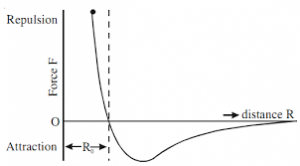## How to Calculate and Solve for Force of Attraction or Repulsion | Magnetic FieldThe image above represents force of attraction or repulsion.

To compute for force of attraction or repulsion, five essential parameters are needed and these parameters are Magnetic Permeability of Vacuum (μo), Strength of Magnetic Pole 1 (m1), Strength of Magnetic Pole 2 (m2), Distance Seperating the Poles (r) and Relative Magnetic Permeability (μR).

The formula for calculating force of attraction or repulsion:

F = μom1m2 / 4πμRr²

Where:

F = Force of Attraction or Repulsion
μo = Magnetic Permeability of Vacuum
m1 = Strength of Magnetic Pole 1
m2 = Strength of Magnetic Pole 2
r = Distance Separating the Poles
μR = Relative Magnetic Permeability

Let’s solve an example;
Find the force of attraction or repulsion when the magnetic permeability of vaccum is 1.2566371E-6, strength of magnetic pole 1 is 10, strength of magnetic pole 2 is 12, distance seperating the poles is 8 and the relative magnetic permeability is 14.

This implies that;

μo = Magnetic Permeability of Vacuum = 1.2566371E-6
m1 = Strength of Magnetic Pole 1 = 10
m2 = Strength of Magnetic Pole 2 = 12
r = Distance Separating the Poles = 8
μR = Relative Magnetic Permeability = 14

F = μom1m2 / 4πμRr²
F = 0.0000012566371(10)(12) / 4π(14)(8)²
F = 0.000150796452 / 4π(14)(64)
F = 0.000150796452 / 11259.468
F = 1.339e-8

Therefore, the force of attraction or repulsion is 1.339e-8.